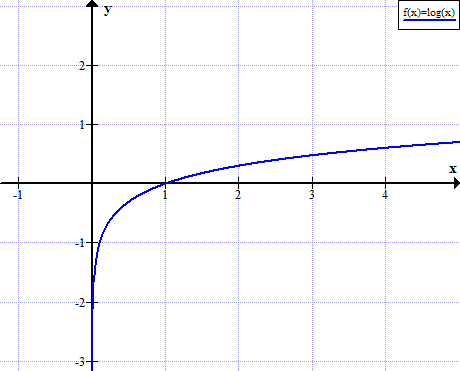# Graph of log(x)

log(x) function graph. Logarithm graph.

y = f (x) = log10(x)### log(x) graph properties

• log(x) is defined for positive values of x.
• log(x) is not defined for real non positive values of x.
• log(x)<0 for 0<x<1
• log(x)=0 for x=1
• log(x)>0 for x>1

Logarithm rules ►